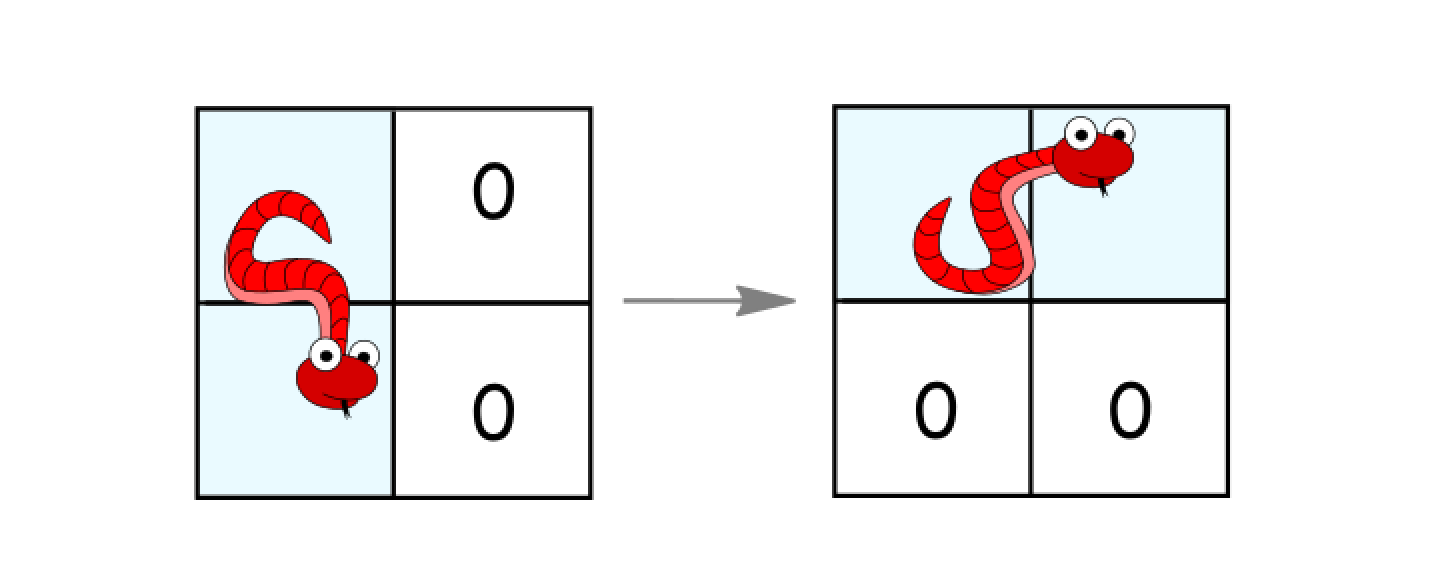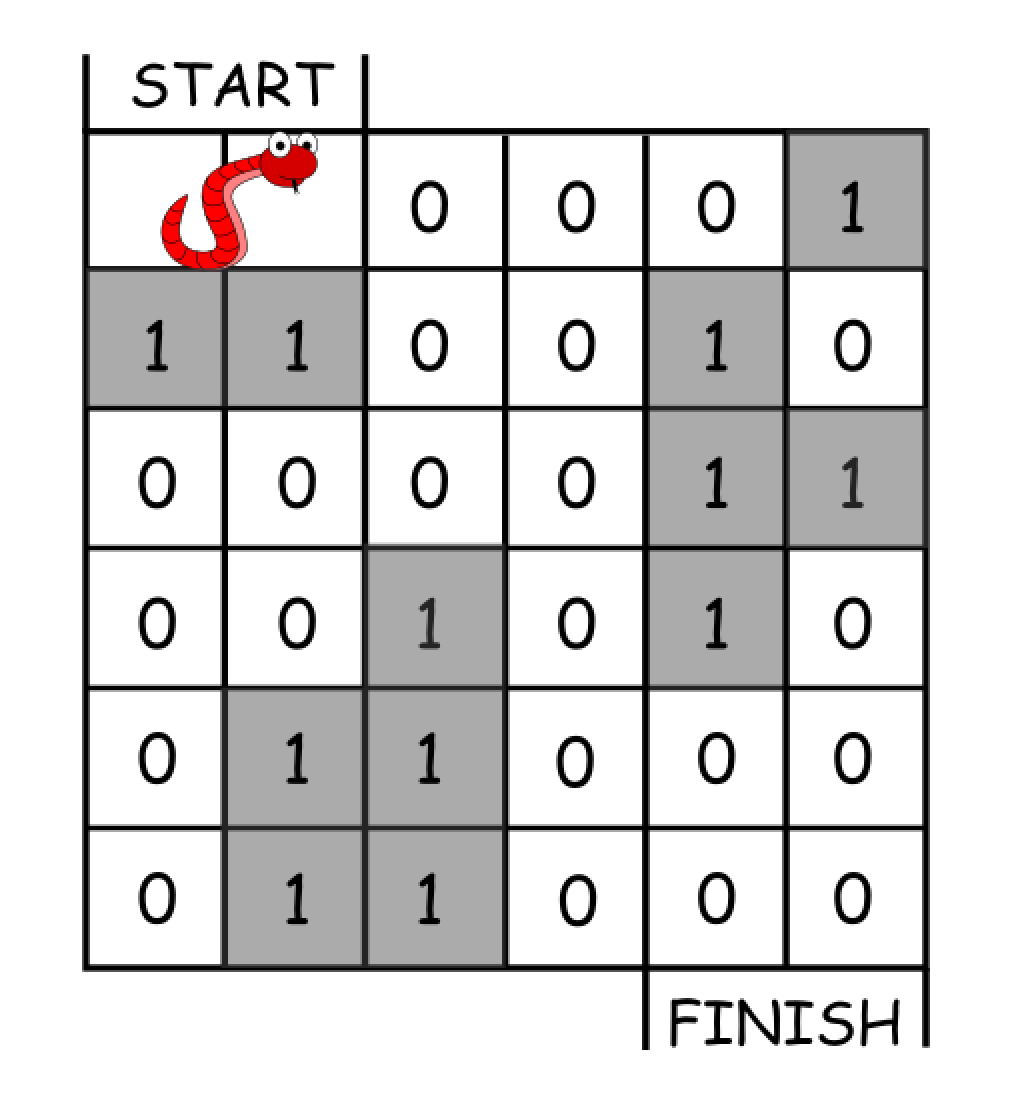# 1210. Minimum Moves to Reach Target with Rotations

In an `n*n` grid, there is a snake that spans 2 cells and starts moving from the top left corner at `(0, 0)` and `(0, 1)`. The grid has empty cells represented by zeros and blocked cells represented by ones. The snake wants to reach the lower right corner at `(n-1, n-2)` and `(n-1, n-1)`.

In one move the snake can:

• Move one cell to the right if there are no blocked cells there. This move keeps the horizontal/vertical position of the snake as it is.
• Move down one cell if there are no blocked cells there. This move keeps the horizontal/vertical position of the snake as it is.
• Rotate clockwise if it’s in a horizontal position and the two cells under it are both empty. In that case the snake moves from `(r, c)` and `(r, c+1)` to `(r, c)` and `(r+1, c)`.• Rotate counterclockwise if it’s in a vertical position and the two cells to its right are both empty. In that case the snake moves from `(r, c)` and `(r+1, c)` to `(r, c)` and `(r, c+1)`.Return the minimum number of moves to reach the target.

If there is no way to reach the target, return `-1`.

Example 1:``````Input: grid = [[0,0,0,0,0,1],
[1,1,0,0,1,0],
[0,0,0,0,1,1],
[0,0,1,0,1,0],
[0,1,1,0,0,0],
[0,1,1,0,0,0]]
Output: 11
Explanation: One possible solution is [right, right, rotate clockwise, right, down, down, down, down, rotate counterclockwise, right, down].
``````

Example 2:

``````Input: grid = [[0,0,1,1,1,1],
[0,0,0,0,1,1],
[1,1,0,0,0,1],
[1,1,1,0,0,1],
[1,1,1,0,0,1],
[1,1,1,0,0,0]]
Output: 9
``````

Constraints:

• `2 <= n <= 100`
• `0 <= grid[i][j] <= 1`
• It is guaranteed that the snake starts at empty cells.

``````class Solution {
public:
int minimumMoves(vector<vector<int>>& grid) {
int res = 0, n = grid.size();
set<vector<int>> visited{{0, 1, 0}};
queue<vector<int>> q;
q.push({0, 1, 0});
while (!q.empty()) {
for (int i = q.size(); i > 0; --i) {
auto t = q.front(); q.pop();
int x = t, y = t, dir = t;
if (x == n - 1 && y == n - 1 && dir == 0) return res;
if (dir == 0) { // horizontal
if (y + 1 < n && grid[x][y + 1] == 0 && !visited.count({x, y + 1, 0})) { // Move right
visited.insert({x, y + 1, 0});
q.push({x, y + 1, 0});
}
if (x + 1 < n && y > 0 && grid[x + 1][y - 1] == 0 && grid[x + 1][y] == 0) {
if (!visited.count({x + 1, y, 0})) { // Move down
visited.insert({x + 1, y, 0});
q.push({x + 1, y, 0});
}
if (!visited.count({x + 1, y - 1, 1})) { // Rote
visited.insert({x + 1, y - 1, 1});
q.push({x + 1, y - 1, 1});
}
}
} else { // vertical
if (x + 1 < n && grid[x + 1][y] == 0 && !visited.count({x + 1, y, 1})) { // Move down
visited.insert({x + 1, y, 1});
q.push({x + 1, y, 1});
}
if (y + 1 < n && x > 0 && grid[x - 1][y + 1] == 0 && grid[x][y + 1] == 0) {
if (!visited.count({x, y + 1, 1})) { // Move right
visited.insert({x, y + 1, 1});
q.push({x, y + 1, 1});
}
if (!visited.count({x - 1, y + 1, 0})) { // Rotate
visited.insert({x - 1, y + 1, 0});
q.push({x - 1, y + 1, 0});
}
}
}
}
++res;
}
return -1;
}
};
``````

``````class Solution {
public:
int minimumMoves(vector<vector<int>>& grid) {
int res = 0, n = grid.size();
queue<vector<int>> q;
q.push({0, 0, 0}); // 0 is horizontal, 1 is vertial
while (!q.empty()) {
for (int i = q.size(); i > 0; --i) {
auto t = q.front(); q.pop();
int x = t, y = t, dir = t;
if (x == n - 1 && y == n - 2) return res;
if ((grid[x][y] & (dir ? 2 : 4)) != 0) continue;
grid[x][y] |= (dir ? 2 : 4);
if (canGoDown(grid, x, y, dir)) q.push({x + 1, y, dir});
if (canGoRight(grid, x, y, dir)) q.push({x, y + 1, dir});
if (canRotate(grid, x, y)) q.push({x, y, !dir});
}
++res;
}
return -1;
}
bool canGoDown(vector<vector<int>>& grid, int x, int y, int dir) {
int n = grid.size();
if (dir == 0) return x + 1 < n && (grid[x + 1][y] & 1) == 0 && (grid[x + 1][y + 1] & 1) == 0;
return x + 2 < n && (grid[x + 2][y] & 1) == 0;
}
bool canGoRight(vector<vector<int>>& grid, int x, int y, int dir) {
int n = grid.size();
if (dir == 0) return y + 2 < n && (grid[x][y + 2] & 1) == 0;
return y + 1 < n && (grid[x][y + 1] & 1) == 0 && (grid[x + 1][y + 1] & 1) == 0;
}
bool canRotate(vector<vector<int>>& grid, int x, int y) {
int n = grid.size();
return x + 1 < n && y + 1 < n && (grid[x + 1][y] & 1) == 0 && (grid[x][y + 1] & 1) == 0 && (grid[x + 1][y + 1] & 1) == 0;
}
};
``````

Github 同步地址:

https://github.com/grandyang/leetcode/issues/1210

https://leetcode.com/problems/minimum-moves-to-reach-target-with-rotations/

https://leetcode.com/problems/minimum-moves-to-reach-target-with-rotations/discuss/392872/C%2B%2B-BFS

https://leetcode.com/problems/minimum-moves-to-reach-target-with-rotations/discuss/393511/JavaPython-3-25-and-17-liner-clean-BFS-codes-w-brief-explanation-and-analysis.

LeetCode All in One 题目讲解汇总(持续更新中…)

 微信打赏Venmo 打赏×

Help us with donation